Mobile QR Code1. (System Integrated Circuit Design Lab, Inha University, 100, Inha-ro, Michuhol-gu, Incheon, Incheon 22212, Korea)

CMOS, pulse amplitude modulation (PAM), pulse width modulation (PWM), low power, transceiver

## I. INTRODUCTION

Recently, the demand for high-speed data transmission has increased rapidly with the development of AI deep-learning autonomous vehicles based on camera sensors.

For high-speed data transmission, serial links are preferred over parallel links due to low power consumption and cost. But high-speed data transmission in serial links is limited by channel bandwidth, which is essentially a low-pass characteristic . Therefore, for high-speed data transmission within a given channel bandwidth, a pulse modulation scheme that increases the number of transmission bits per symbol is used in serial links.

The most common pulse modulation scheme in high-speed serial links is a PAM-X. As shown in Fig. 1(a) and (b), PAM-X is a pulse modulation that increases the number of differential levels (X) and reduces the symbol rate by $\log _{2}X$ times compared to binary signaling (PAM-2). However, for a given SNR satisfaction, the full output swing of the signal must be increased, which causes the power consumption of the transmitter output driver to be increased .

Another pulse modulation scheme is a PWM-X. As shown in Fig. 1(c), PWM-X increases the number of bits per symbol by increasing the number of falling edges (X). In other words, a PWM-X signal has a rising edge and a falling edge per symbol. So, unlike a PAM-X, a clock and data recovery (CDR) is replaced by a phase-locked loop (PLL) in the receiver . In addition, a PWM-X always uses two differential levels, which is why the power consumption of the PWM-X transceiver is lower than that of PAM-X. Also, a PAM driver is implemented with a current mode logic (CML), but a PWM driver is implemented with a CMOS logic. Therefore, PWM-X improves power efficiency by technology scaling more than PAM-X. However, an increase in the number of falling edges (X) leads to a decrease in the minimum pulse width, causing an increase in inter-symbol interference (ISI) induced by channel loss.

For power efficiency improvement and high data rate, the dual-mode PAM-10 scheme was introduced as shown in Fig. 1(d) . This scheme can reduce the power consumption of the transmitter output driver by decreasing the number of differential levels (X) through common mode modulation. Also, the dual-mode PAM-10 scheme ensures the same symbol rate as PAM-16. However, many the number of differential levels (X=10) still require high supply voltage. Also, the dual-mode PAM-10 employs the static output driver. Therefore, its power efficiency improvement by technology scaling may be hard compared to PWM-X used on a CMOS logic.

For the reduction of pin-count and high-speed data transmission, the conventional PWAM scheme was introduced as shown in Fig. 1(e) . This scheme uses only 5 differential levels compared to the existing 4-bit/symbol PAM-X (i.e., dual-mode PAM-10  and PAM-16 ). So, the conventional PWAM scheme can reduce the power consumption of the transmitter output driver. However, PWM-4 restricts the minimum pulse width to $\frac{8}{7}T_{b}$, which is similar to PAM-2, thus limiting high-speed data transmission.

In summary, PAM-X, PWM-X, dual-mode PAM-10 and conventional PWAM have restrictions on high-speed data transmission or power efficiency improvement by technology scaling. Therefore, we propose a novel PWAM signaling scheme as shown in Fig. 1(f) to achieve high data rate transmission and power efficiency improvements by technology scaling simultaneously.

This paper is organized as follows: in Section II, the proposed PWAM signaling scheme is presented and compared to the conventional 4-bit/symbol pulse modulation scheme. the transceiver implementation of the proposed scheme is described in Section II. Section III shows the simulation results of the 10-Gb/s transceiver designed in a 180 nm CMOS process for power efficiency verification, and Section IV concludes.

##### Fig. 1. Waveforms of various pulse modulations: (a) PAM-2; (b) PAM-4; (c) PWM-4; (d) dual-mode PAM-10; (e) conventional PWAM (PWM-4 and PAM-4); (f) proposed PWAM (dual-mode PAM-4 and PWM-2).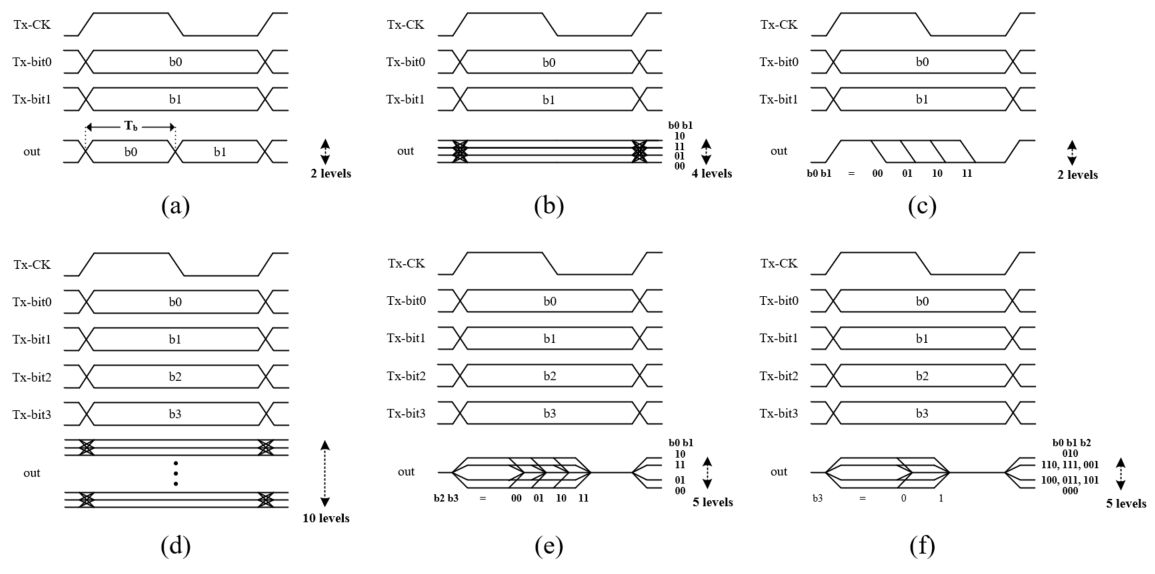## II. PROPOSED PWAM SCHEME

### 1. Proposed PWAM Signaling

The proposed PWAM signaling transmits 4-bits per symbol in a combination of dual-mode PAM-4 and PWM-2. As shown in Fig. 2, the dual-mode PAM-4 uses both common-mode and differential-mode, unlike PAM-X, which employs only differential-mode. Consequently, the dual-mode PAM-4 scheme can modulate 3-bit data to eight differential levels through three common levels $\left(V_{cm2},V_{cm1},V_{cm0}\right)$. In other words, it has the same transmission capability as PAM-8. For that reason, the proposed PWAM scheme can change PWM-4, employed in the conventional PWAM scheme, to PWM-2. Also, the minimum pulse width of the proposed PWAM scheme is increased.

As shown in Fig. 3(a), since the differential levels of $V_{cm2}$ and $V_{cm0}$ overlap the differential levels of $V_{cm1}$, the number of differential levels (X) of the proposed PWAM scheme are 5 including a zero level for PWM-2. That is, the number of differential levels (X) is decreased compared to the 4-bit/symbol pulse modulation scheme (i.e., dual-mode PAM-10  and PAM-16 ), thereby reducing power consumption. In addition, PWM-2 drivers based on CMOS logic help further improve power efficiency by technology scaling.

The important features for the proposed PWAM signaling and comparisons with 4-bit/symbol pulse modulation schemes can be summarized as follows.

1) The proposed PWAM scheme improves the minimum pulse width to $1.5T_{b}$ compared to the conventional PWAM scheme. And the inter-symbol interference (ISI) induced by channel loss is reduced. This is due to a combination of dual-mode PAM-4 and PWM-2. In this work, the falling edge of the proposed PWAM signal is synchronized to CLK-135 and CLK-225. So, the minimum pulse width becomes three over eights for the 1-unit interval of the proposed PWAM signal. And assuming that the 1-unit interval of a 1-bit/symbol PAM-2 is 1$T_{b}$, the 1-unit interval of the 4-bit/symbol proposed scheme is 4$T_{b}$. Therefore, the minimum pulse width ($T_{p}$) of the proposed PWAM signal is calculated as follows.

$T_{p}=\frac{3}{8}\mathrm{UI}_{\text{proposed}\,\,\text{scheme}}=\frac{3}{8}\times 4T_{b}=1.5T_{b}$

2) The proposed PWAM scheme has an increased SNR compared to dual-mode PAM-10  and PAM-16 . This is because it uses only 5 differential levels compared to the other 4-bit/symbol pulse modulation schemes mentioned above.

3) Compared to dual-mode PAM-10  and PAM-16 , the power consumption of the transceiver can be reduced and the power efficiency by technology scaling can be further improved. This is possible because the proposed PWAM scheme has fewer differential levels (X=5) and a 1-bit PAM driver is replaced by a 1-bit PWM driver compared to the other 4-bit/symbol pulse modulation schemes mentioned above.

4) Since PWM-2 has a rising edge and a falling edge for each symbol, the clock can be recovered by a PLL instead of a CDR in the receiver and an 8B10B encoder for CDR is not required in the transmitter. That is, PWM-2 simplifies the circuits for clock recovery in PAM-X.

5) Under a lossy channel environment, the differential-mode is a dominant factor for BER performance than the common-mode. The minimum pulse width of the common-mode is $4T_{b}$, which is larger than that (=$1.5T_{b}$) of the differential-mode. This means, When the voltage difference between adjacent levels in the differential-mode and the voltage difference between adjacent levels in the common-mode is the same, the ISI of the differential-mode is greater than the ISI of the common-mode. Therefore, under a lossy channel environment, the BER is determined by the differential-mode.

Fig. 4 shows a block diagram of the proposed PWAM transceiver. In the transmitter, Tx-PLL generates multi-phased Tx-CLKs required for serial to parallel converter and PWM driver as an external reference clock (REF CLK). The serial to parallel converter converts serial data into 4-bit parallel data (Tx-bit0, Tx-bit1, Tx-bit2 and Tx-bit3) through multi-phased Tx-CLKs. As shown in Fig. 4, only Tx-bit3 is modulated with PWM signal (Tx-PWM) by the PWM driver, and the remaining 3-bit parallel data (Tx-bit0, Tx-bit1 and Tx-bit2) and Tx-PWM is processed by the PAM encoder for dual-mode PAM operation. Then, the PAM driver generates the proposed PWAM signal as an output of the PAM encoder. In the receiver, the reference clock (Rx-REF CLK) is extracted from the proposed PWAM signal by CLK sampler, and it is recovered by Rx-PLL for generating multi-phased Rx-CLKs. The flash ADC detects the differential-mode PAM, common mode PAM and PWM using the recovery clocks (Rx-CLKs) and threshold voltages, and it determines the thermometer codes. Then, the thermometer codes are converted or recovered to 4-bit parallel data (Rx-bit0, Rx-bit1, Rx-bit2, and Rx-bit3) by the decoder.

##### Fig. 2. Single-ended waveform of dual-mode PAM-4: (a) 2-differential levels at $V_{cm2}$ case; (b) 4-differential levels at $V_{cm1}$ case; (c) 2-differential level at $V_{cm0}$ case.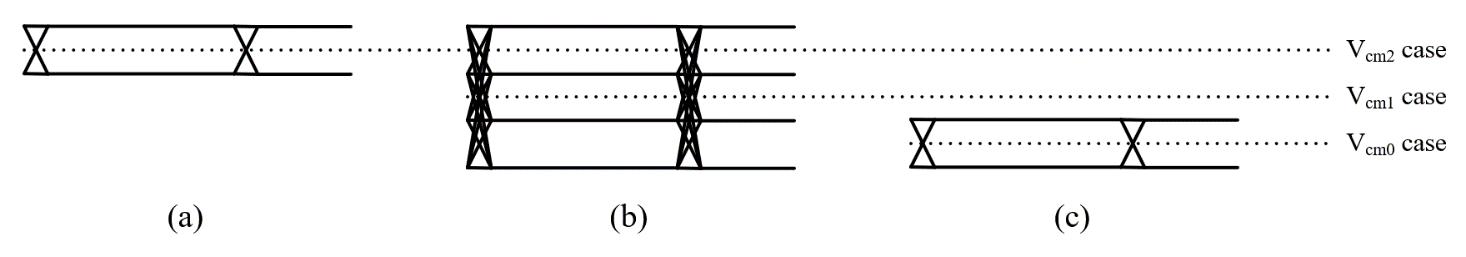##### Fig. 3. The proposed PWAM (dual-mode PAM-4 and PWM-2) format: (a) differential-mode; (b) common-mode.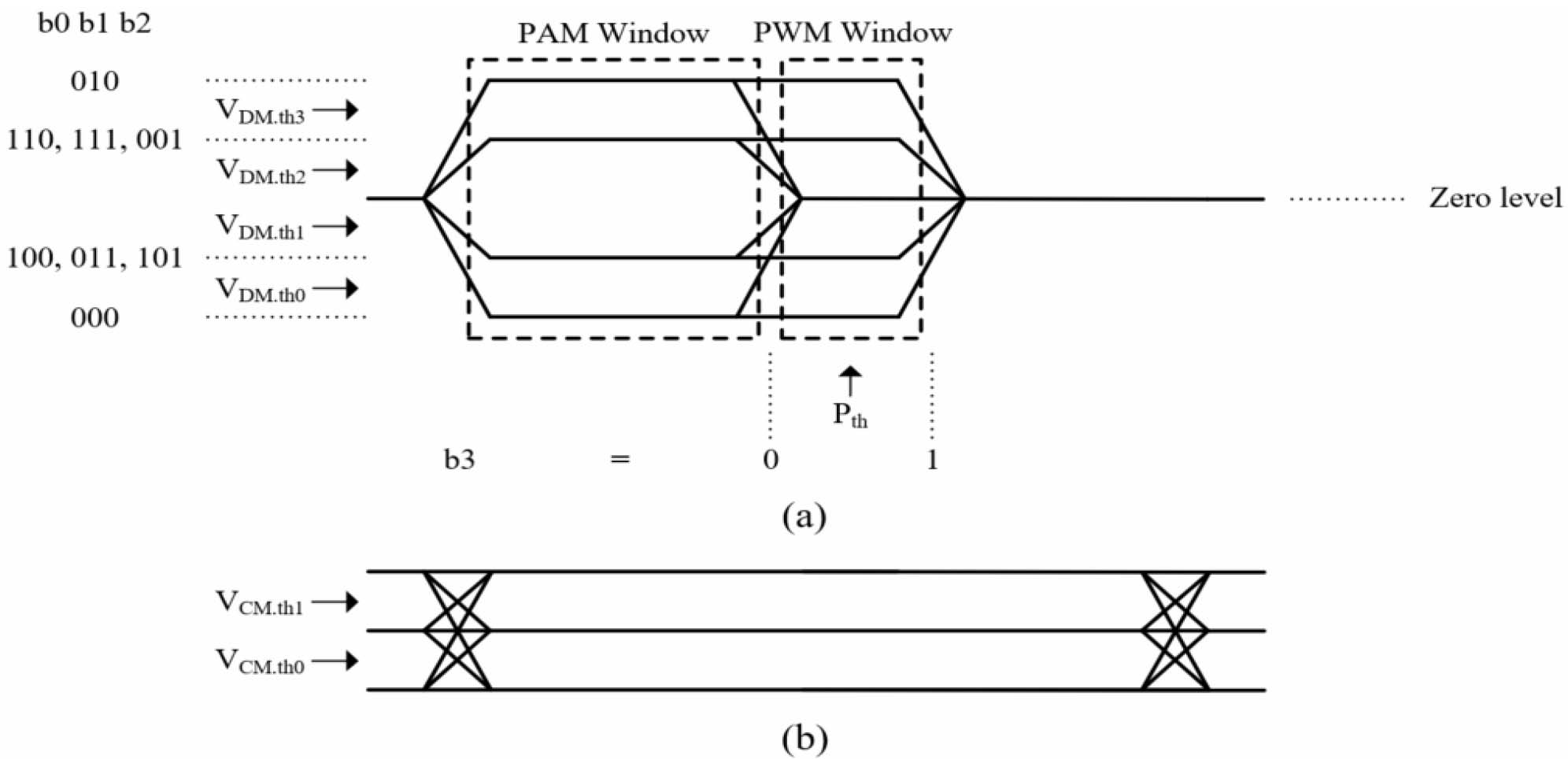##### Fig. 4. The proposed PWAM (dual-mode PAM-4 and PWM-2) transceiver block diagram.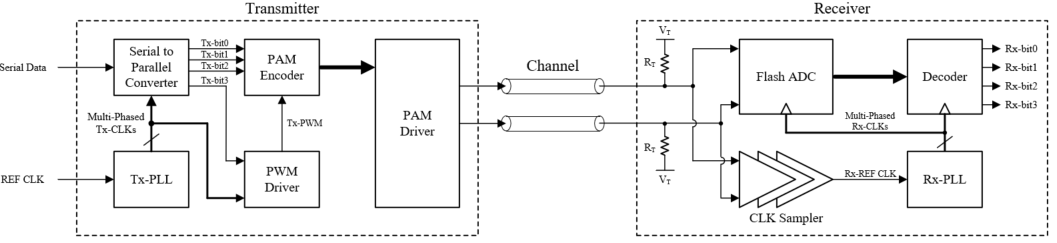### 2. Transmitter Architecture and Design

As shown in Fig. 4, the transmitter consists of Tx-PLL, serial to parallel converter, PWM driver, PAM encoder, and PAM driver.

As shown in Fig. 5, Tx-PLL is based on a conventional charge pump phase-locked loop (CPPLL), and it includes a phase frequency detector (PFD), a charge pump (CP), a low-pass filter (LPF), a voltage-controlled oscillator (VCO), a duty cycle corrector (DCC), and divider. DCC and four-stage differential ring VCO are employed in Tx-PLL for the exact phase of eight multi-phased Tx-CLKs. If a 45-degree phase difference between eight multi-phased Tx-CLKs is not guaranteed, a bit error may occur due to serial to parallel converter and PWAM demodulation, and the minimum pulse width of 1.5$T_{b}$ cannot be guaranteed. Therefore, the DCC shown in Fig. 5 and four-stage differential ring VCO are designed for Tx-PLL. In this work, 10-Gb/s serial data is transmitted, so the Tx-PLL must generate a 2.5 GHz clock through an external reference clock (REF CLK).

The serial to parallel converter is a circuit that converts serial data into 4-bit parallel data (Tx-bit0, Tx-bit1, Tx- bit2 and Tx-bit3). If REF CLK is synchronized with serial data, serial data can be converted into 4-bit parallel data by 4-different phase clocks with a 90-degree difference. Fig. 6 shows the block diagram of the serial to parallel converter, indicating that the first stage flip-flops sample the serial data into parallel data through 4-different phase clocks with a 90-degree difference, and that the parallel data is synchronized to CLK-0 at the second stage flip-flops. In this work, an extended-true single phase clock (E-TSPC) flip flop was used for the serial to parallel converter, and it features high-speed operation, lower power consumption, and smaller area due to the fewer number of transistors than the conventional TSPC flip-flop .

As shown in Fig. 7, PWM driver consists of a phase selector and a phase combiner . In the phase selector, NMOS transistors on the left determine the rising edge of Tx-PWM, and the phase combiner maintains the value of Tx-PWM at ‘1 for a while, and then NMOS transistors on the right of the phase selector decide the falling edge of Tx-PWM. As shown in Fig. 8, in order for the Tx-PWM signal to have one rising edge and two different falling edges for 1 unit interval, its rising edge is synchronized to CLK-0, and its falling edges are determined by CLK-135 or CLK-225. In this work, if Tx-bit3 is ‘0’, the falling edge of Tx-PWM is synchronized with CLK-135. And if Tx-bit3 is ‘1’, it is synchronized with CLK-225. Thus, CLK-180 can be used as a threshold phase ($P_{th}$) for demodulating bit3 information in the receiver. Also, the phase difference of 1$T_{b}$ between CLK-135 and CLK-225 becomes the sampling time margin for demodulating Tx-bit3 in the receiver.

The PAM encoder is a circuit for making the minimum pulse width of common-mode 4Tb as shown in Fig. 3(b), and its truth table is listed in Table 1. Also, the PAM encoder is shown in Fig. 9 and it is designed with CMOS logic to improve power efficiency by technology scaling. The overall behavior of the PAM encoder is as follows: 1) the common-mode decision circuit determines $V_{cm}$<2:0> from Tx-bit<2:0>. This is to pick up a common level among three common levels $(V_{cm2},V_{cm1},$ $V_{cm0})$. 2) the encoder generates all differential-mode outputs of S<6:0> and Sb<5:0> when Tx-PWM is '1', and all common-mode outputs of S<6:0> and Sb<5:0> when Tx-PWM is '0'. The outputs of each mode are listed in Table 1. 3) in the 3 to 1 MUX array, differential-mode outputs and common-mode outputs corresponding to the common level are selected among all outputs from the encoder. 4) in flip-flop array, the selected differential-mode outputs and common-mode outputs are sampled by Tx-PWM. 5) in the 2 to 1 MUX array, when Tx-PWM is '1', S<6:0> and Sb<5:0> becomes the selected differential-mode outputs, and when Tx-PWM is '0', S<6:0> and Sb<5:0> becomes the selected common-mode outputs. This is to sustain the common level when the differential level is zero level.

The PAM driver is designed with a current mode logic (CML) and employs current steering topology for stable current source operations [2,6]. As shown in Fig. 10, PAM driver consists of left, center, and right current sources for the dual-mode PAM operation. The left current sources drive 2I, so it is a driver for $V_{cm2}$. The center and the left current sources together drive 6I, so they are drivers for $V_{cm1}$, and NMOS transistors for S<6> are added for current steering topology when the common-mode is $V_{cm2}$. Lastly, the right current sources drive 10I together with the left and the center current sources, so they are drivers for $V_{cm0}$. In addition, the current sources of the PAM driver are designed as a cascode current source for stable current when the common level is changed.

The differential output (OUTP - OUTN) and common output ([OUTP + OUTN]/2) by S<6:0> and Sb<5:0> in the PAM driver are summarized in Table 1, which uses the gray-code mapping method. This is to ensure one-bit error between adjacent differential outputs .

##### Table 1. Truth table for PAM encoder and PAM driver output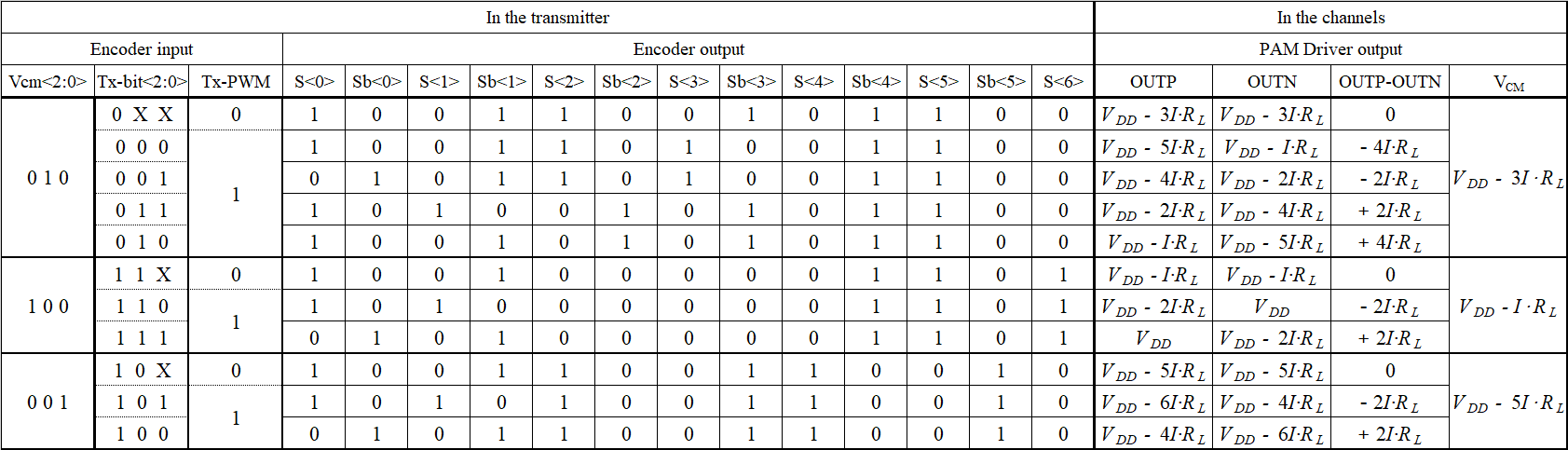##### Fig. 5. Tx-PLL based on a conventional charge pump phase-locked loop.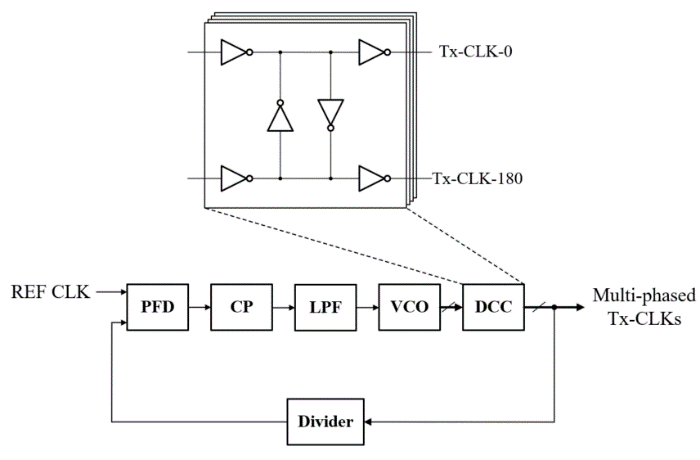##### Fig. 6. Serial to parallel converter.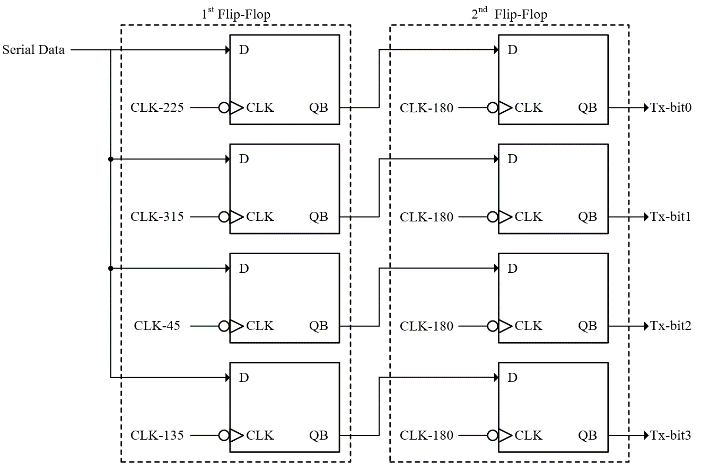##### Fig. 7. PWM driver based CMOS logic.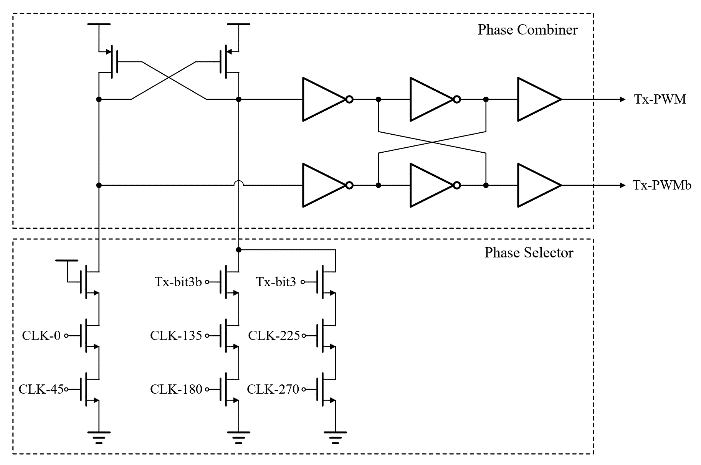##### Fig. 8. PWM signal (Tx-PWM) modulated by Tx-bit3.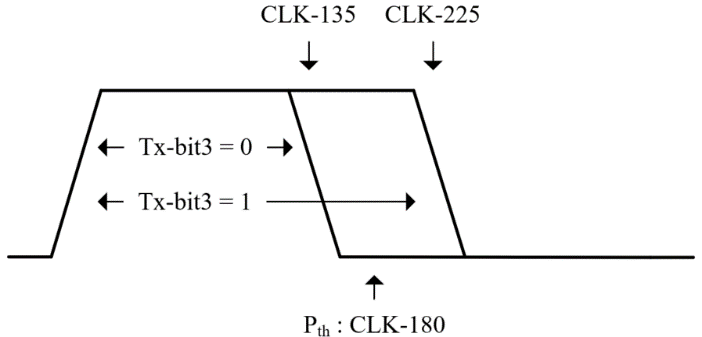### 3. Receiver Architecture and Design

The receiver consists of CLK sampler, Rx-PLL, flash ADC, and decoder including retimer, as shown in Fig. 4.

The CLK sampler is a circuit for extracting Rx-REF CLK from the proposed PWAM signal and consists of CM blocking circuit, continuous time linear equalizer (CTLE), variable gain amplifier (VGA), and PWM sampler, as shown in Fig. 11.

Since the conventional differential amplifier cannot perform common-mode rejection for high-frequency common-mode voltage , CM blocking circuit is required. For example, if the high-frequency common-mode of the proposed PWAM signal is input to the conventional differential amplifier, the gate-source voltage ($V_{GS}$) of the NMOS differential pair cannot be fixed. In that case, the drain current ($I_{D}$) of the NMOS differential pair becomes unstable and causes a ripple in the common-mode voltage. After all, since it means that the bias of the circuit is unstable, the RC-degenerated differential pair  and PWM sampler based on the conventional differential amplifier cannot be worked properly. However, when the CM blocking circuit based on the CTLE with negative resistance and capacitance  is designed as $I_{SS1}<I_{SS2}$, its common-mode voltage is generated by $I_{SS2}$ driven by DC bias rather than $I_{SS1}$ driven by high-frequency common-mode. For that reason, compared to the conventional differential amplifier, the ripple of the common-mode voltage can be reduced, and the high-frequency common-mode of the proposed PWAM signal can be blocked. Therefore, in order for the circuit based on the conventional differential amplifier to work properly, the CM blocking circuit must be the first stage of the CLK sampler.

As shown in Fig. 11, the CTLE designed as $I_{SS1}>I_{SS2}$ becomes the second stage of the CLK sampler to suppress the ISI induced by channel loss, and VGA is followed to compensate for the signal amplitude reduced by the CM blocking circuit.

The PWM sampler, the last stage of the CLK sampler, extracts the reference clock (Rx-REF CLK) from the differential-mode of the proposed PWAM signal. In addition, Fig. 12 shows the operation process of the PWM sampler through only amplification and digital operations without any feedback topology, and its operation process is as follows: 1) The differential amplifier with cross-coupled PMOS load and resistor load amplifies the differential input so that one of the positive and negative signals is at a level below the inverter logic threshold. 2) The amplified positive and negative signals are inverted with the inverters. 3) When performing XOR operation on the inverted positive signal and the negative signal, the reference clock (Rx-REF CLK) is extracted from the proposed PWAM signal. Meanwhile, under a lossy channel environment, the reference clock (Rx-REF CLK) may include data-dependent jitter, so the jitter should be filtered by Rx-PLL.

As shown in Fig. 13, Rx-PLL has a structure similar to that of the Tx-PLL. Also, a four-stage differential ring VCO and DCC are employed in the Rx-PLL to demodulate the proposed PWAM signal without the occurrence of bit error. However, the divider is excluded to generate a full-rate clock. And a variable delay circuit (VDC) is added to minimize the phase difference between the rising edge of the proposed PWAM signal and the rising edge of the recovered clock (Rx-CLK0). Assuming that the phase offset of Rx-PLL is the value of '0', the phase difference is caused by the delay ($\Delta T$) of the CLK sampler as shown in Fig. 4. If it is not minimized, a bit error may occur during the demodulation of dual-mode PAM-4 and PWM-2. Therefore, to minimize the phase difference, a method in which recovered clocks (Rx-CLKs) is delayed by the time for $1\mathrm{UI}-\Delta T$ is used. That is, as shown in Fig. 13, VDC should be designed to have a delay of $1\mathrm{UI}-\Delta T$. Additionally, since a conventional CPPLL has low-pass characteristics with respect to the input reference clock , the bandwidth of the Rx-PLL should be narrowly set to filter data-dependent jitter of the reference clock (Rx-REF CLK), and high-order low-pass filter (LPF) should be considered.

As shown in Fig. 14, the flash ADC determines the thermometer codes from the proposed PWAM signal to recover 4-bit parallel data, and it consists of a differential-mode PAM demodulator, a common-mode PAM demodulator and a PWM demodulator. The differential-mode PAM demodulator detects the differential-mode level with three threshold voltages ($\mathrm{V}_{\mathrm{DM},\mathrm{th}0},0,\,\,\mathrm{V}_{\mathrm{DM},\mathrm{th}3}$) shown in Fig. 3(a), and it determines the three thermometer codes ($\mathrm{T}_{\mathrm{DM}}$<2:0>). Also, in order for the differential-mode PAM demodulator to operate in the PAM window, it should be operated by Rx-CLK90. The common-mode PAM demodulator detects th3.e common-mode level with two threshold voltages ($\mathrm{V}_{\mathrm{CM}.\mathrm{th}0},\,\,\mathrm{V}_{\mathrm{CM}.\mathrm{th}1}$) shown in Fig. 3(b), and it decides the two thermometer codes ($\mathrm{T}_{\mathrm{CM}}$<1:0>). Also, the common-mode PAM demodulator should be operated by Rx-CLK180 which is aligned at the center timing of the common-mode signal. The PWM demodulator detects the PWM signal with two threshold voltages ($\mathrm{V}_{\mathrm{DM},\mathrm{th}1},\,\,\mathrm{V}_{\mathrm{DM},\mathrm{th}2}$) and a threshold phase ($\mathrm{P}_{\mathrm{th}}$) shown in Fig. 3(a), and it determines the two thermometer codes ($\mathrm{T}_{\text{PWMP}},\,\,\mathrm{T}_{\text{PWMN}}$). In order to demodulate Rx-bit3 information, the PWM demodulator should be operated by Rx-CLK180 which is the threshold phase ($\mathrm{P}_{\mathrm{th}}$). In addition, the slicer employed in the flash ADC is the track and regenerate slicer , which can be operated at higher speeds than the strong-arm slicer.

The decoder converts the output codes of the flash ADC ($\mathrm{T}_{\mathrm{DM}}$<2:0>, $\mathrm{T}_{\mathrm{CM}}$<1:0>, $\mathrm{T}_{\text{PWMP}}$ and $\mathrm{T}_{\text{PWMN}}$) into binary codes, and it is implemented with standard CMOS logic and the truth table of Table 2. Then, the four retimers recover the binary codes, and their outputs become 4-bit parallel data (Rx-bit0, Rx-bit1, Rx-bit2 and Rx-bit3).

In this work, the threshold voltages required in the demodulators is generated by a resistor ladder, and each threshold voltage level is as follows: three threshold voltages for differential-mode PAM ($\mathrm{V}_{\mathrm{DM},\mathrm{th}0},0,\,\,\mathrm{V}_{\mathrm{DM},\mathrm{th}3}$) are $-3I\cdot R_{L},0,+3I\cdot R_{L},$ two threshold voltages for common-mode PAM ($\mathrm{V}_{\mathrm{CM}.\mathrm{th}0},\,\,\mathrm{V}_{\mathrm{CM}.\mathrm{th}1}$) are $V_{DD}-2I\cdot R_{L},$ $V_{DD}-4I\cdot R_{L}$, and two threshold voltages for PWM ($\mathrm{V}_{\mathrm{DM},\mathrm{th}1},\,\,\mathrm{V}_{\mathrm{DM},\mathrm{th}2}$) are $-I\cdot R_{L},\,\,I\cdot R_{L}$.

##### Table 2. Truth table for the decoder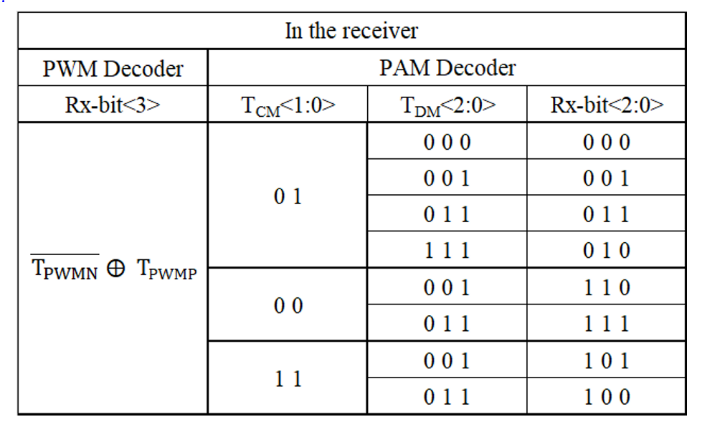##### Fig. 11. CLK sampler: CM blocking circuit, CTLE, VGA, PWM sampler.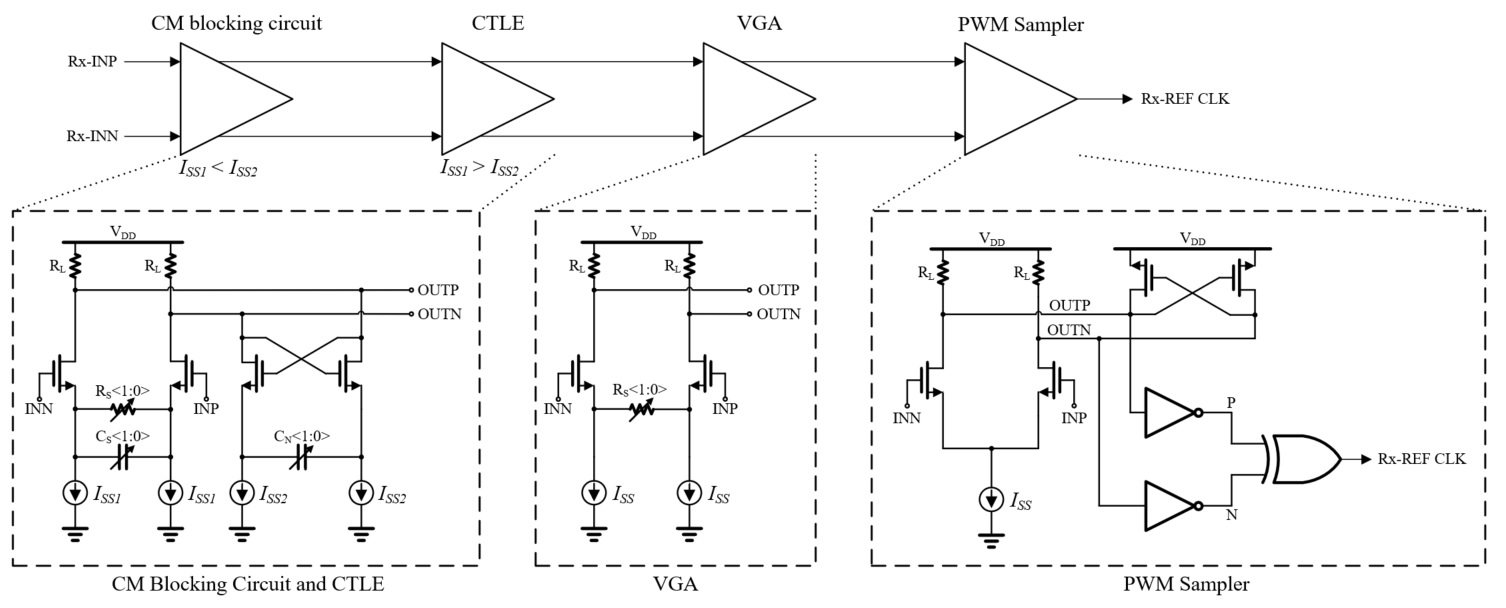##### Fig. 12. Timing diagram for PWM sampler##### Fig. 13. Rx-PLL based on a conventional charge pump phase-locked loop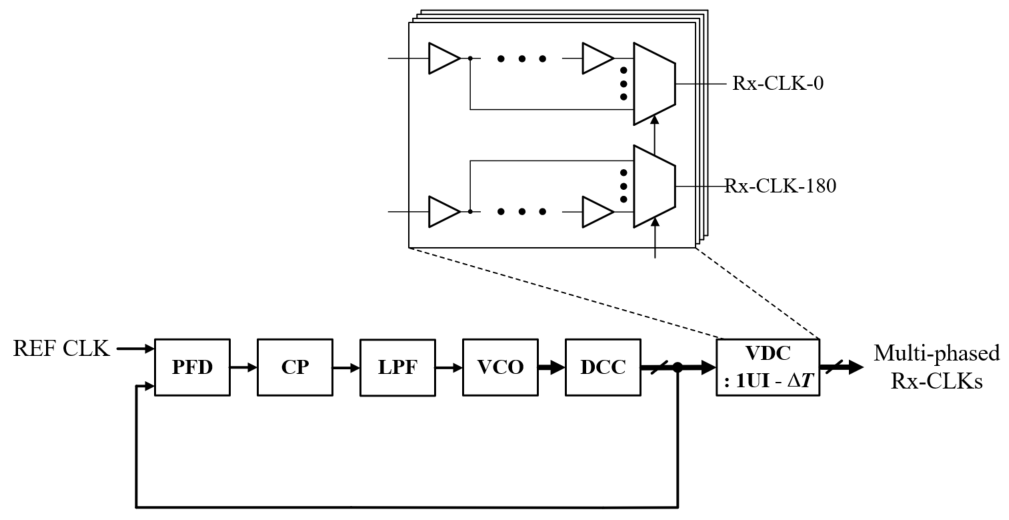##### Fig. 14. Flash ADC: differential mode PAM demodulator, common mode PAM demodulator, PWM demodulator.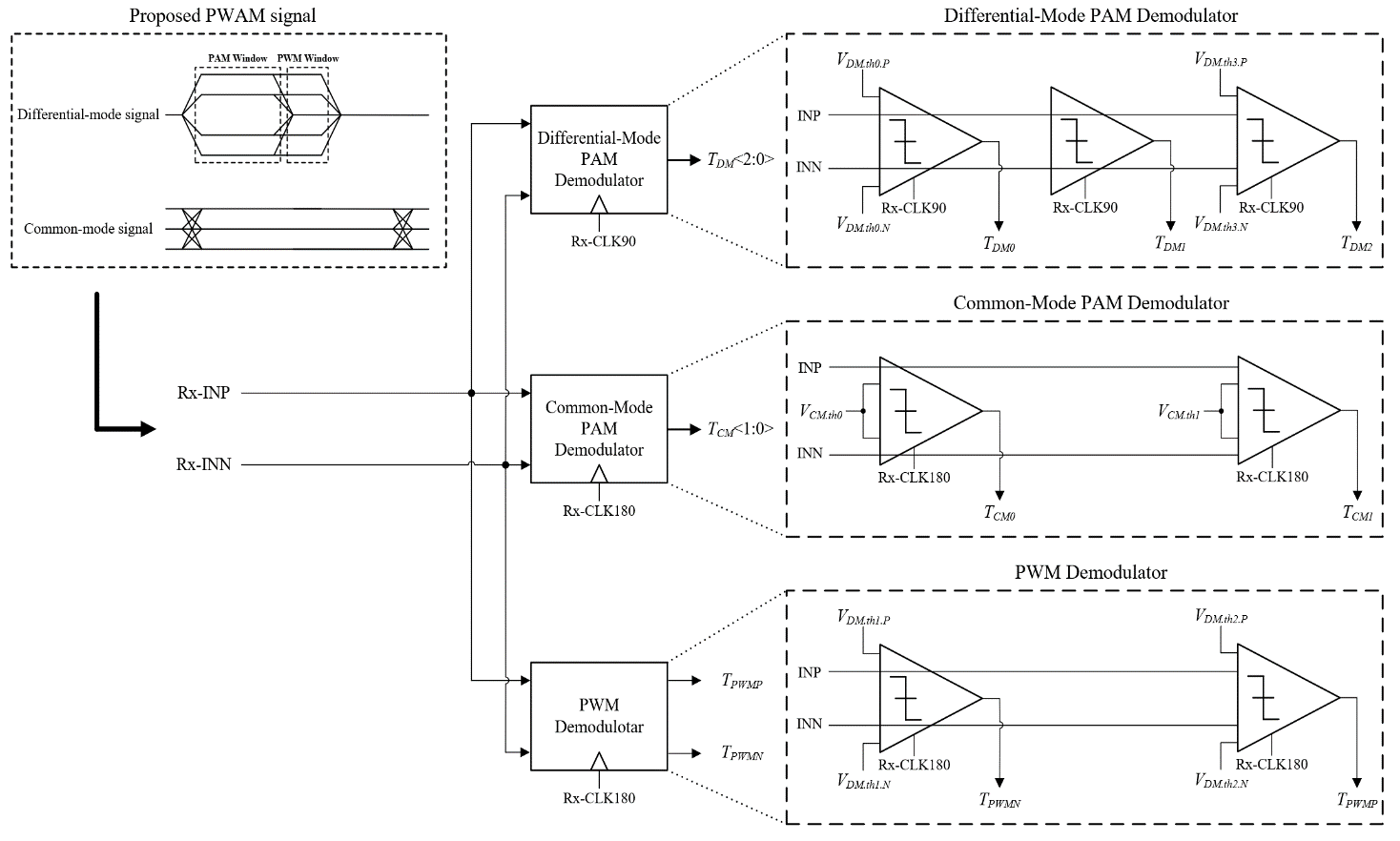## III. SIMULATION RESULTS

To verify the power efficiency of the proposed PWAM signaling scheme, the 10-Gb/s transceiver was designed in a 180 nm CMOS process. In addition, FR4 type 315 mm channel was used for verification, and PRBS31's 10-Gb/s serial data and 250 MHz external reference clock (REF CLK) were applied to the transmitter inputs.

Fig. 15 shows the simulated S21 of the channel to verify. In this work, the proposed transceiver is designed to target 10Gb/s. So, the differential-mode frequency of the proposed PWAM signal is approximately 3.34 GHz and the channel loss at that frequency is -6.08 dB. Also, the common-mode frequency of the proposed PWAM signal is 1.25 GHz and the channel loss at that frequency is -2.72 dB. That is, since the minimum pulse width ($=1.5T_{b}$) of the differential-mode is shorter than that ($=4T_{b}$) of the common-mode, the channel loss of the differential-mode has a relatively large value compared to that of the common mode.

Fig. 16 shows the simulated Tx-PWM signal eye-diagram. The duty cycle of the Tx-PWM signal is 38.2\% or 64\%, and it verifies that the Tx-PWM signal is modulated by Tx-bit3. Also, the peak-to-peak jitter of Tx-PWM is 5.02 ps.

Fig. 17 shows the simulated eye-diagram of the transmitter output. And it shows the differential-mode and common-mode are generated by the PAM driver, and the voltage difference (${\Delta}$V) between each adjacent level is approximately 200 mV. In addition, Fig. 17(a) shows that the differential-mode is synchronized to the Tx-PWM signal. Meanwhile, the glitch shown in Fig. 17(b) may occur due to the operation of 2 to 1 MUX array to make the minimum pulse width of the common-mode 4$T_{b}$. And the glitch appearing in common-mode causes an unstable zero level shown in Fig. 17(a). However, since the glitch is a very high-frequency component of 30 GHz or higher, it can be filtered by the channel. As shown in Fig. 18(b), the glitch is suppressed by channel loss. So, as shown in Fig. 18(a), the unstable zero level induced by the glitch rarely appears in the differential-mode of the receiver input. 18(a). That is, the unstable zero level does not affect the middle eye and BER.

Fig. 18 shows the simulated eye-diagram of the receiver input. Due to the channel loss of 6.08 dB at 3.34 GHz, the voltage difference (${\Delta}$V) in PAM window is approximately 100 mV. Also, the voltage difference (${\Delta}$V) in common-mode is approximately 124 mV due to a channel loss of 2.72 dB at 1.25 GHz. That is, it is larger than the voltage difference (${\Delta}$V) of differential-mode. Therefore, this analysis shows that, under a lossy channel environment, differential-mode operation is more critical for BER performance than common-mode operation.

Fig. 19 shows the simulated eye-diagram for common-mode voltage of CM blocking circuit. Because of the CM blocking circuit, the high-frequency common-mode of the proposed PWAM signal rarely appears in the output node of the CM Blocking circuit. In other words, it can be blocked.

Fig. 20 shows the simulated eye-diagram of Rx-REF CLK. And it shows that Rx-REF CLK can be extracted by only amplification and digital operations without any feedback system, and the simulated peak-to-peak jitter of Rx-REF CLK is 51.82 ps.

The simulated eye-diagram of recovered clock (Rx-CLK0) is shown in Fig. 21, and the simulated peak-to-peak jitter of Rx-CLK0 is 12.53 ps. Since the PLL removes the jitter for the input reference clock , Rx-CLK0 has a smaller jitter compared to the jitter of Rx-REF CLK. Additionally, Fig. 21 shows that the phase difference between the differential-mode PWAM signal and Rx-CLK0 is almost '0' by VDC having a delay of $1\mathrm{UI}-\Delta T$.

Among the four-bit recovered data (Rx-bit0, Rx-bit1, Rx-bit2 and Rx-bit3), the eye-diagram of Rx-bit0 is shown in Fig. 22. The simulated peak-to-peak jitter of the recovered data (Rx-bit0) is 11.52 ps.

In this work, the supply voltage of the transceiver is 1.8 -V, and equalization is not applied for better power efficiency. However, for Rx-REF CLK extraction, a small equalization block was inserted in the CLK sampler.

The transmitter for 10-Gb/s serial data transmission consumes 134 mW in a 180 nm CMOS process. The Tx-PLL, the serial to parallel converter, the PWM driver, the PAM encoder, and the PAM driver consume 16.26 mW, 4.43 mW, 16.39 mW, 24.2 mW, and 72.72 mW, respectively. the receiver consumes 95 mW. The CLK sampler, the Rx-PLL, the flash ADC and the decoder consume 32 mW, 34.29 mW, 14.4 mW, and 14.29 mW, respectively. Also, the power consumption for each sub-block in the transmitter and receiver is shown in Fig. 23.

Fig. 24 shows the normalized power consumption of the proposed 10-Gb/s transmitter designed in a 180 nm CMOS process and a 65 nm CMOS process. The PAM driver reduces the power consumption by 1.5 times only by supply voltage reduction without reducing the static current for a fixed output swing. However, the power consumption is reduced by more than 4 times because other circuits, including the PWM driver, are designed with a standard CMOS logic. This analysis means that a standard CMOS logic has a greater reduction in power consumption by technology scaling. This also suggests that the proposed PWAM scheme, which includes PWM-2, over the existing 4-bit pulse modulation schemes (e.g., PAM-16, dual-mode PAM-10) can further improve power efficiency by technology scaling. Meanwhile, to verify the improvement of the power efficiency, the proposed transmitter was also designed in a 65 nm CMOS process.

The simulation results and performance of the transceiver employing the proposed PWAM signaling scheme are summarized in Table 3 and it includes the performance of the transceiver for dual-mode PAM-10 , PWAM , PAM-16 , and PAM-4 [14-16] scheme introduced in the past.

The power consumption of the 10-Gb/s transceiver employing the proposed scheme is 229 mW. Compared to dual-mode PAM-10 , the power consumption of the proposed PWAM transceiver with the same data rate and the same 180 nm CMOS process was reduced by 1.86 times and the power efficiency was improved by 1.86 times. This is because the proposed scheme has fewer differential levels (X=5) than the dual-mode PAM-10 scheme.

To compare other works [13-16] designed in different process, the relative power efficiency of the proposed transceiver ($\mathrm{RPE}$) is defined as

##### (1)
$\mathrm{RPE}=\mathrm{S}\cdot \mathrm{V}\cdot \mathrm{T}\cdot \mathrm{PE}_{\mathrm{Tx}}+\mathrm{S}\cdot \mathrm{V}\cdot \mathrm{PE}_{\mathrm{Rx}}$

where $\mathrm{S}$ is the relative speed rate, $\mathrm{V}$ is the relative supply voltage, $\mathrm{T}$ is '1' if the transmitter driver type of other work is the same current-mode logic (CML) and is one over fours if it is the source-series-terminated (SST) driver, $\mathrm{PE}_{\mathrm{Tx}}$ is the power efficiency of the proposed transmitter, and $\mathrm{PE}_{\mathrm{Rx}}$ is the power efficiency of the proposed receiver. For example, for 64-Gb/s transceiver , S is one over 6.4, V is one over twos, T is one over fours, $\mathrm{PE}_{\mathrm{Tx}}$ is 13.4 pJ/bit, and $\mathrm{PE}_{\mathrm{Rx}}$ is 9.5 pJ/bit. And, to consider the device performance difference between a FinFET process and a CMOS process, V is considered as the low supply voltage among the dual supply voltages of 64-Gb/s transceiver . Therefore, the relative power efficiency of the proposed transceiver ($\mathrm{RPE}$) for 64-Gb/s transceiver  is approximately 1 pJ/bit by Eq. (1), and it is smaller than 2.96 pJ/bit, the power efficiency of 64-Gb/s transceiver  designed in the most advanced process among other works [13-16]. In the same way, the relative power efficiencies of the proposed transceiver for PAM-16 , and PAM-4 [15,16] are 2.23 pJ/bit, 1.98 pJ/bit, and 1.14 pJ/bit, respectively, by Eq. (1). They are smaller than the power efficiencies of 2.38 pJ/bit, 4.92 pJ/bit, and 2.29 pJ/bit of PAM-16  and PAM-4 [15,16]. Therefore, it is suggested that the proposed scheme further improves power efficiency by technology scaling.

To check bit errors in the modulation and demodulation process of the proposed transceiver, the simulation was additionally performed by a delay circuit and an XOR circuit under a noisy power supply environment. If Tx-bits are delayed by a delay circuit having the propagation delay of the transceiver and channel, the delayed Tx-bits will be synchronized with Rx-bits. That is, the bit errors can be confirmed by XOR operating them. The simulation result for checking bit error showed that all four outputs of the XOR circuits showed a value of '0'. Therefore, no bit error occurred during modulation and demodulation of the transceiver.

##### Table 3. Performance summary and comparison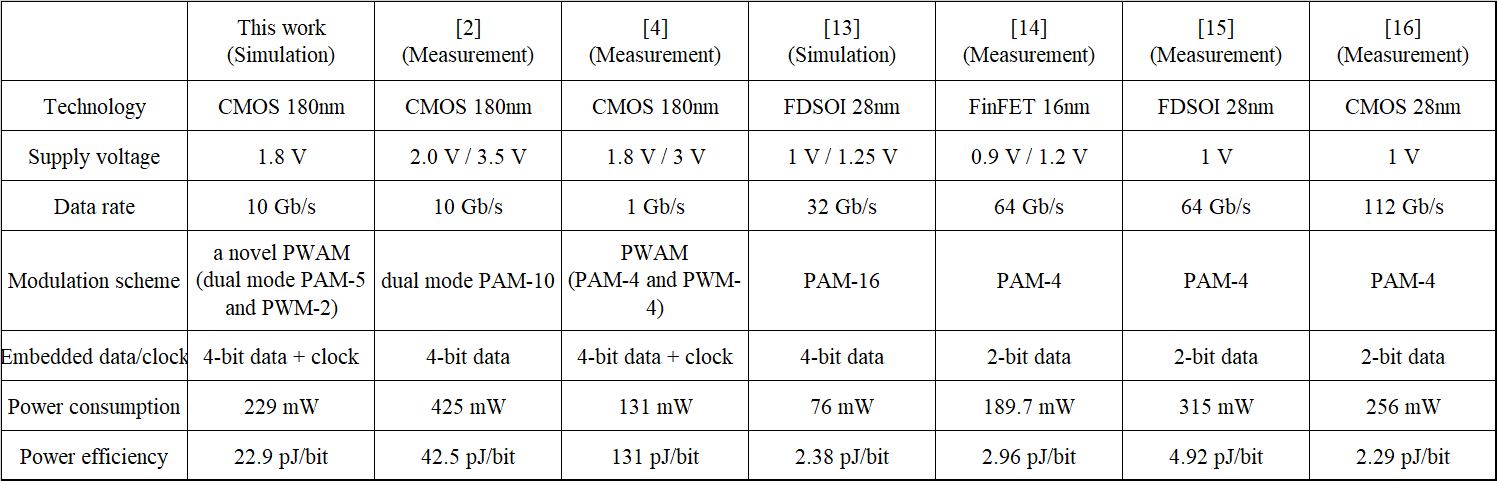##### Fig. 15. Simulated S21 of the channel.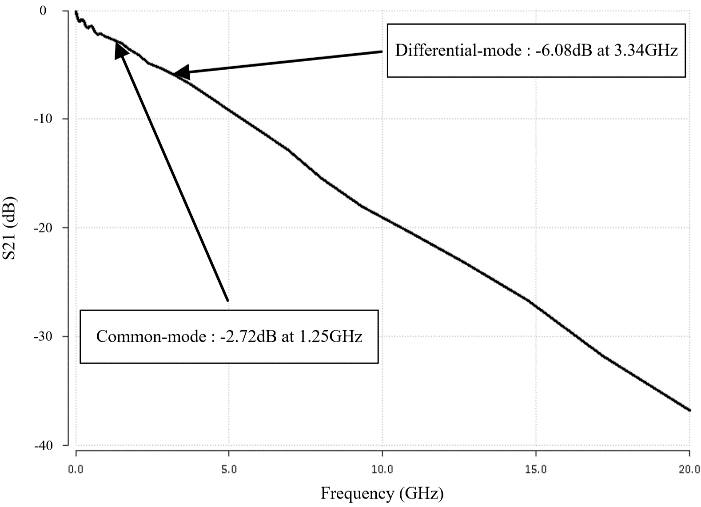##### Fig. 16. Simulated eye-diagram for Tx-PWM signal.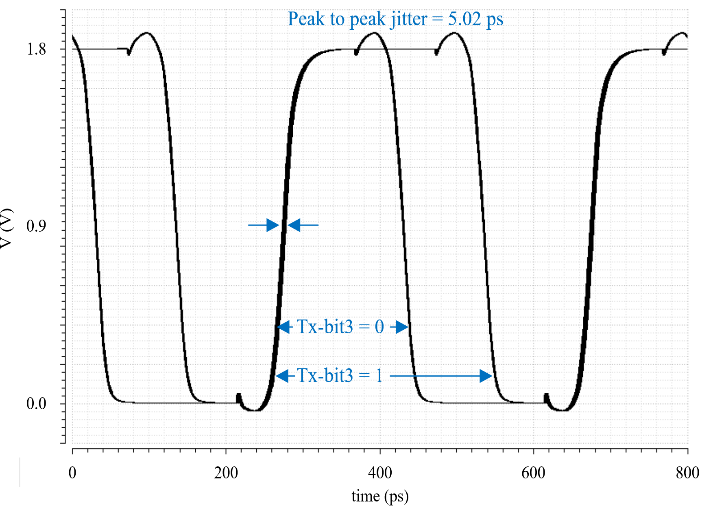##### Fig. 17. Simulated eye-diagram of the transmitter output: (a) differential-mode; (b) common-mode.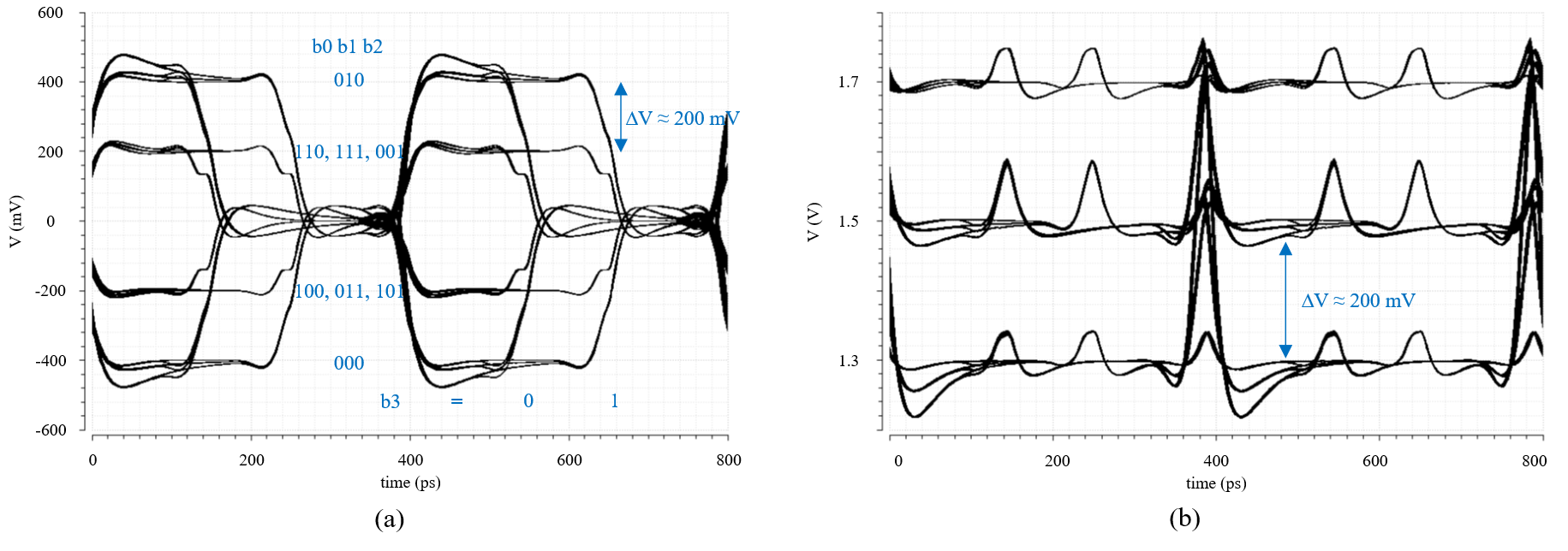##### Fig. 18. Simulated eye-diagram of the receiver input: (a) differential-mode; (b) common-mode.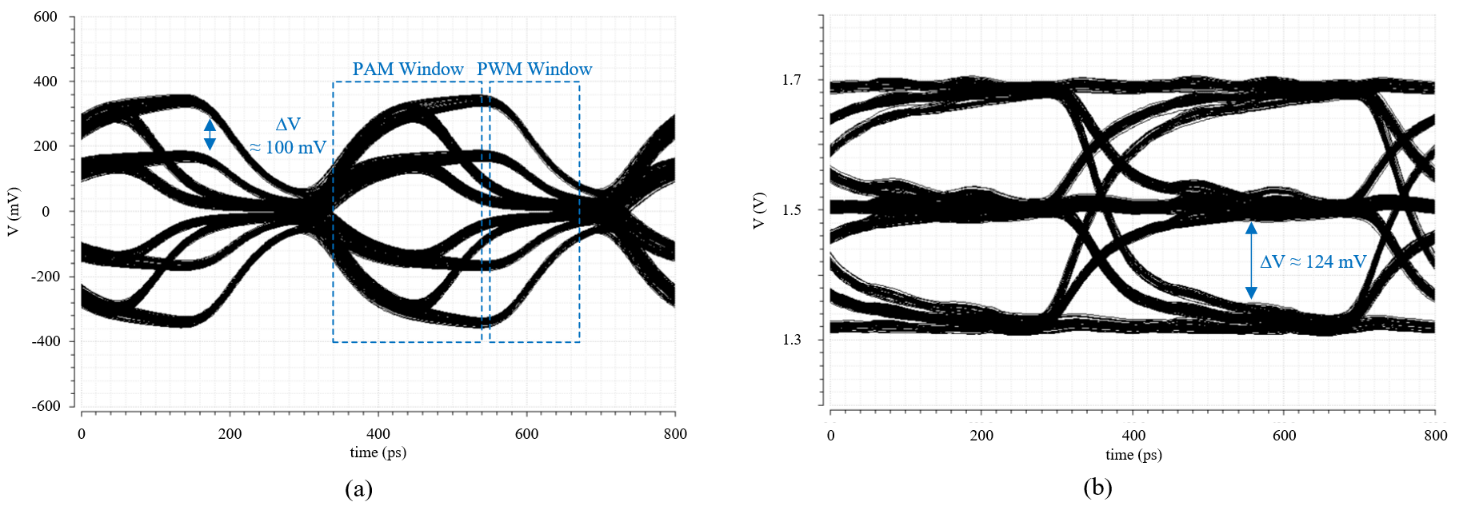##### Fig. 19. Simulated eye-diagram for common-mode voltage of CM blocking circuit.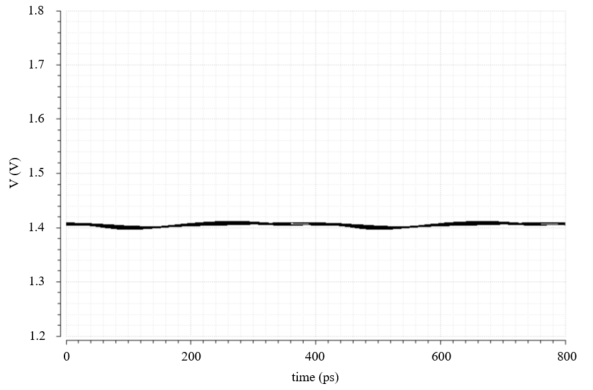##### Fig. 20. Simulated eye-diagram of Rx-REF CLK.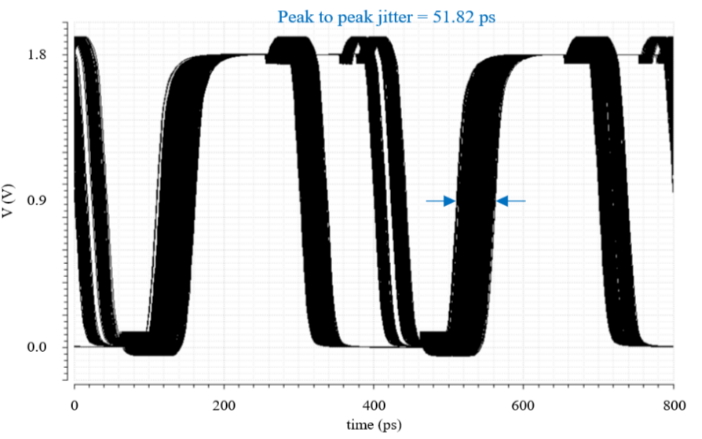##### Fig. 21. Simulated eye-diagram of differential-mode PWAM signal and recovered clock (Rx-CLK0).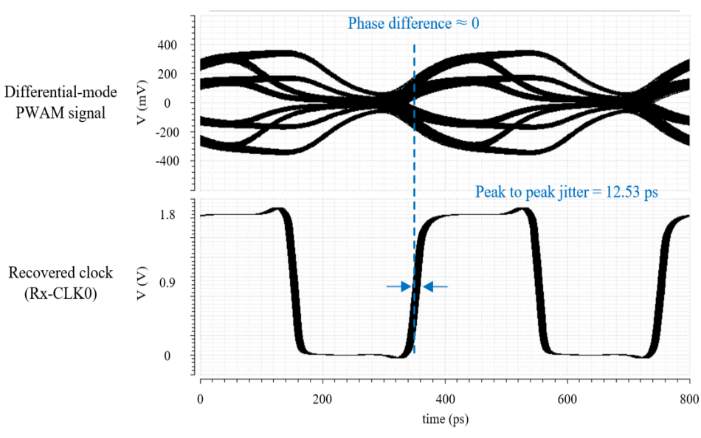##### Fig. 22. Simulated eye-diagram of the recovered data (Rx-bit0).##### Fig. 23. The power consumption for each sub-block: (a) transmitter; (b) receiver.##### Fig. 24. Normalized power consumption of the proposed 10-Gb/s transmitters designed in a 180 nm CMOS process and a 65 nm CMOS process.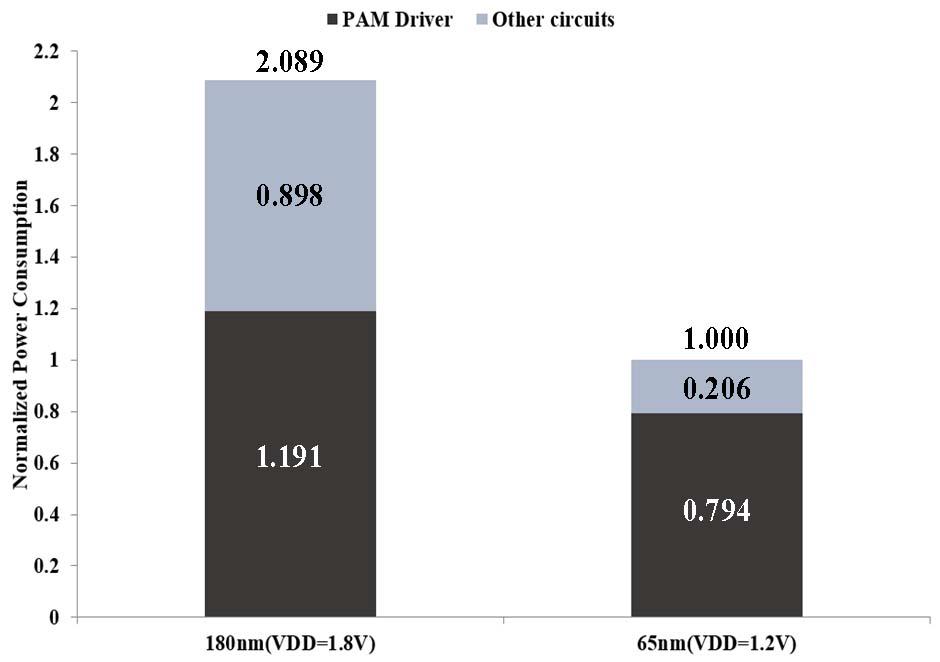## IV. CONCLUSIONS

This paper proposed a novel PWAM signaling scheme, which combines a dual mode PAM-4 and a PWM-2. The proposed scheme improves the insufficient minimum pulse width of the conventional PWAM to enable high-speed data transmission. In addition, since the 4-bit/symbol proposed scheme uses only 5 differential levels compared to the existing 4-bit/symbol PAM scheme (e.g., PAM-16, dual-mode PAM-10), the power consumption of the transceiver can be reduced. Also, due to PWM-2, the proposed scheme further can improve power efficiency by technology scaling.

## ACKNOWLEDGMENTS

This research was supported by the National Research Foundation of Korea (NRF) (No.2020R1F1A1077088), National R&D Program through the National Research Foundation of Korea (NRF) funded by Ministry of Science and ICT (No. 2020M3H2A1076786), and the MSIT (Ministry of Science and ICT), Korea, under the ITRC (Information Technology Research Center) support program (IITP-2021-0-02052) supervised by the IITP (Institute for Information & Communications Technology Planning & Evaluation). Authors also thank the IDEC program and for its hardware and software assistance for the design and simulation.

## References

1
Granberg T., 2004, Handbook of Digital Techniques for High-Speed Design, Englewood Cliffs, NJ: Prentice Hall PTR2
Song B., Kim K., Lee J., Burm J., Feb. 2013, A 0.18 ${\mu}$m CMOS 10- Gb/s Dual-Mode 10-PAM Serial Link Transceiver, Circuits and Systems I, IEEE Transactions on, Vol. 60, No. 2, pp. 457-4683
Chen W.-H., Dehng G.-K., Chen J.-W., Liu S.-I., Oct. 2001, A CMOS 400-Mb/s serial link for AS-memory systems using a PWM scheme, Solid-State Circuits, IEEE Journal of, Vol. 36, No. 10, pp. 1498-15054
Yang C.-Y., Lee Y., May. 2008, A PWM and PAM Signaling Hybrid Technology for Serial-Link Transceivers, Instrumentation and Measurement, IEEE Transcations on, Vol. 57, No. 5, pp. 1058-10705
Jung M., Fuhrmann J., Ferizi A., Fischer G., Weigel R., Ussmueller T., Dec. 2011, Design of a 12 GHz Low-Power Extended True Single Phase Clock (E-TSPC) Prescaler in 0.13${\mu}$m CMOS technology, Microwave Conference 2011, 2011. APMC 2011. IEEE Asia-Pacific, Vol. 5, No. 8, pp. 1238-1241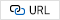6
Cheng H., Musa F. A., Carusone A. C., Aug. 2009, A 32/16-Gb/s Dual-Mode Pulsewidth Modulation Pre-Emphasis (PWM-PE) Transmitter With 30-dB Loss Compensation Using a High-Speed CML Design Methodology, Circuits and System I, IEEE Transacations on, Vol. 56, No. 8, pp. 1794-18067
Farjad-Rad R., Yang C.-K. K., Horowitz M. A., Lee T. H., May. 1999, A 0.4- ${\mu}$m CMOS 10-Gb/s 4-PAM pre-emphasis serial link transmitter, Solid-State Circuits, IEEE Journal of, Vol. 34, No. 5, pp. 580-5858
Razavi B., 2001, Design of Analog CMOS Integrated Circuits, New York: McGraw-Hill9
Gondi S., Razavi B., Sep. 2007, Eqaulization and Clock and Data Recovery Techniques for 10-Gb/s CMOS Serial-Link Receivers, Solid-State Circuits, IEEE Journal of, Vol. 42, No. 9, pp. 1999-201110
Lim B., Yoo C., Nov. 2017, A 12-Gb/s Continuous-time Linear Equalizer with Offset Canceller, Semiconductor Technology and Science, IEIE Journal of, Vol. 19, No. 2, pp. 220-22611
Gardner F. M., 2005, Phaselock Techniques, 3$^{\mathrm{rd}}$ ed. Hoboken12
Chen K. -C., Kuo W. W. -T., Emami A., Mar. 2021, A 60- Gb/s PAM4 Wireline Receiver With 2-Tap Direct Decision Feedback Equalization Employing Track-and-Regenerate Slicer in 28-nm CMOS, Solid-State Circuits, IEEE Journal of, Vol. 56, No. 3, pp. 750-76213
Celik F., Akkaya A., Leblebici Y., Feb. 2021, A 32 Gb/s PAM-16 Tx and ADC-Based Rx AFE with 2-tap embedded analog FFE in 28 nm FDSOI, Microelectronics Journal, Vol. 108, pp. Aritcle 10496714
Wang L., Fu Y., LaCroix M., Chong E., Carusone A. C., Mar. 2018, A 64Gb/s PAM-4 transceiver utilizing an adaptive threshold ADC in 16nm FinFET, Solid-State Circuits, IEEE International Coference on, pp. 110-11115
Depaolio E., et al. , Jan. 2019, A 64 Gb/s Low-Power Transceiver for Short-Reach PAM-4 Electrical Links in 28-nm FDSOI CMOS, Solid-State Circuits, IEEE Journal of, Vol. 54, No. 1, pp. 6-1716
Ye B., et al , Feb. 2022, A 2.29pJ/b 112Gb/s Wireline Transceiver with RX 4-Tap FFE for Medium-Reach Applications in 28nm CMOS, Solid-State Circuits, IEEE International Coference on, pp. 118-119HwanUng Kim received the B.S. degree in Electronic Engineering from Inha University, Incheon, South Korea, in 2021. He is currently pursuing the M.S degree in Electrical and Computer Engineering with Inha University. His research interests include PLL, CDR, high-speed serial interface, and transceiver design for PAM/PWM signaling

Jin-Ku Kang received the Ph.D. degree in electrical and computer engineering from North Carolina State University, Raleigh, NC, USA. From 1983 to 1988, he was with Samsung Electronics, Inc., South Korea, where he was involved in memory and ASIC design. In 1988, he was with Texas Instruments, South Korea. From 1996 to 1997, he was with Intel Corp., Portland, OR, USA, as a Senior Design Engineer, where he was involved in high-speed I/O and timing circuits for microprocessors. Since 1997, he has been with Inha University, Incheon, South Korea, where he is currently a professor and leads the System IC Design Laboratory in the Department of Electronics Engineering. His research interests include high-speed/low-power mixed-mode circuit design for high-speed serial interfaces.